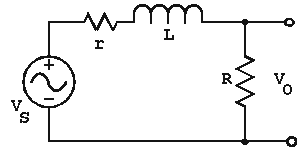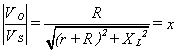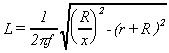the Technology Interface/Fall 96

# A Simple Procedure for Measuring Inductance

by
Guillermo Rico
gurico@nmsu.edu
Department of Engineering Technology
New Mexico State University

This method requires the use of a signal generator and an analog or digital multimeter.

1. Measure the wire resistance of the inductor under measurement; call it r.

2. Assemble the following circuit using a known resistance R between 100 S and 1 KS. Adjust the generator to a frequency of 2 to 10 KHz, sine wave, and 1.00 volt rms. The voltmeter must be connected across points A and B, that is, reading the voltage from the generator.3. Move the voltmeter test leads across points C and B and take down the reading; call it x.

4. Substitute your values into the following formula to determine the inductance L.CIRCUIT ANALYSISUsing voltage division, the output voltage can be expressed asLet x be the magnitude of the transfer ratio. Then,If we use a source voltage of 1.00 V, then x will be equivalent in magnitude to the output voltage VO. Therefore, we simply have to solve for XL in order to obtain the inductance value in terms of x, resistance values, and frequency.Square root both sides and replace XL by 2BfL to obtainIf you have questions for Dr. Rico click on his name.